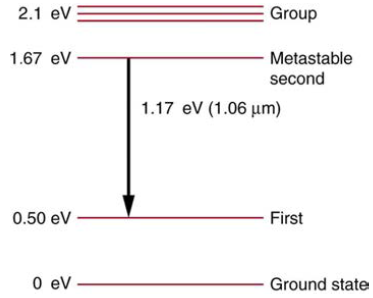Chapter 30, Problem 34PE

Chapter
Section
Textbook Problem

Some of the most powerful lasers are based on the energy levels of neodymium in solids, such as glass, as shown in Figure 30.65. (a) What average wavelength light can pump the neodymium into the levels above its metastable state? (b) Verify that the 1.17 eV transition produces 1.06 μm radiation.Figure 30.65 Neodymium atoms in glass have these energy levels, one of which is metastable. The group of levels above the metastable state is convenient for achieving a population inversion, since photons of many different energies can be absorbed by atoms in the ground state.

To determine

(a)

The average wavelength light can pump the neodymium into the levels above its metastable state.

Explanation

Given Data:

Given energy level diagram of lasers based on energy levels of neodymium in solids such as glass.

Formula Used:

Energy is given as

E=hcλ

Where, E= Energy

h= Planck's Constant

c= Speed of light

λ= Wavelength

Energy released during transition between metastable state and ground state is given as

ΔE=EmEg

Where ΔE= Energy released during the transition between two states

Em= Energy of metastable state

Eg= Energy of ground state

Calculation:

From energy level diagram,

Emabove=2.1eV

Eg=0eV

Energy released during transition from ground state to above metastable state is calculated as

ΔE=(2.1eV)(0eV)

ΔE=2.1eV

Now, wavelength emitted by laser is calculated using Planck's equation

We have h=6.626×1034J.s

E=2.1eV

c=3×108m/sec

Substituting the values in equation of energy, we have

E=hcλ

2.1eV=(6.626× 10 34J

To determine

(b)

The 1.17eV transition produces 1.06μm radiation.

Still sussing out bartleby?

Check out a sample textbook solution.

See a sample solution

The Solution to Your Study Problems

Bartleby provides explanations to thousands of textbook problems written by our experts, many with advanced degrees!

Get Started

Absorption of the majority of nutrients takes place across the mucus-coated lining of the stomach. T F

Nutrition: Concepts and Controversies - Standalone book (MindTap Course List)

Why do radio telescopes have relatively poor resolving power?

Horizons: Exploring the Universe (MindTap Course List)

What is a chemical reaction?

Biology: The Dynamic Science (MindTap Course List)